Alternative Interpretation of the Lorentz-transformation | OMICS International
Journal of Physical Mathematics

# Alternative Interpretation of the Lorentz-transformation

Deyssenroth H*

Senior Researcher, Germany

*Corresponding Author:
Deyssenroth H
Senior Researcher, Germany
Tel: 49- 762187175
E-mail: [email protected]

Received Date: October 12, 2015; Accepted Date: October 28, 2015; Published Date: November 10, 2015

Citation: Deyssenroth H (2015) Alternative Interpretation of the Lorentz-transformation. J Phys Math 7:149. doi:10.4172/2090-0902.1000149

Copyright: © 2015 Dmitriev AL. This is an open-access article distributed under the terms of the Creative Commons Attribution License, which permits unrestricted use, distribution, and reproduction in any medium, provided the original author and source are credited.

Visit for more related articles at Journal of Physical Mathematics

#### Abstract

The Lorentz-Transformation (LT) is the basis of the Theories of Relativity, which are capable of describing the experimentally manifold confirmed relativistic phenomena that deviate from classical physics. Here I present a proof that results in an alternative interpretation of the LT. In particular, the LT cannot be applied to high relative velocities and related space-time modeling – one of the most important tools in physics and astronomy – and will lead to a dead end. Two experiments are proposed to test this idea.

#### Keywords

Lorentz-transformation; Doppler effect; Frequency; Galilean transformation

#### Introduction

The relativistic doppler effect

Suppose two reference frames A and B with identical emitters and detectors move with constant velocities against each other, but their velocities against a fixed point are not known. Due to the measured change of frequency, observers in those systems could calculate the relative velocity between them. However, in classical physics a formula for this model does not exist.

If an observer in A can assume that he is at rest and B moves with velocity –v in his direction, then the classical Doppler-formula  is valid (with β=v/c).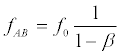(1)

This formula is not based on a transmission medium like air for sound. It is sufficient to assume a constant velocity in relation to a reference point outside of this test system.

Alternatively, if system B is at rest, and the observer in A moves to B with the velocity +v then a different Doppler-formula is valid.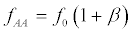(2)

This is observed that outside of frames A and B where the information is transmitted with constant velocity c, independent of the movements of A and B.

Take for example standing on a hill and consider frequency changes observed by drivers (A and B) in moving cars emitting sound at a given frequency in a valley.

Does the observer in B arrive at the same conclusion as the observer in A?

fBA=fAB? (3)

fBB=fAA? (4)

No. If A and B have different velocities vA and vB in reference to a fixed point, the observers will measure different frequencies despite the relative velocity v being the same for both. This is a consequence of (1) and (2) being scaled differently.

If the velocities vA and vB are not known, one has to estimate the relative velocity v. The best approximation is the mean value of the frequencies fAB and fAA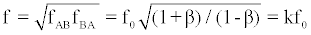(5)

This is the formula of the Relativistic Doppler effect . k is the Bondi-k-Calculus factor from which all formulas of the special theory of relativity (STR) can be derived [3-4]

According to this formula, the observed frequencies are symmetric and identical for A and B. This is a mathematical method to compensate the unknown absolute velocities of A and B. It is valid for all information-transmissions, including water waves and light, given the correct constant transmission velocity c. There is no physical mechanism included in this formula.

The extension of formula (5) with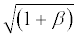yields for the boost in x-direction (y'=y, z'=z)

f=f0 ɣ(1+β) (6)

and extended with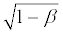f=(f0/ɣ)(1- β) (7)

These are the classical Doppler formulas but now with a correction factor ɣ that compensates the lack of knowledge of the absolute velocities of A and B. It is based on the geometric mean of the observations in A and B.

ɣ=1/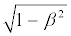(8)

We regard the binomial expression (1-β2)=(1+β) (1-β):

The opposite signs of β do not belong to the system B that moves back and forth towards A. This results in the consideration of the simultaneous movement of A and B to or from each other with the relative velocity v. The factor ɣ is the geometric mean of these velocity proportions.

If A and B stipulate to send mutual N pulses within their local time T0 then the equation (7) becomes

f=N/T=(N/T0)/ɣ(1-β) (9)

T=T0ɣ (1-β)=ɣ(T0-x0 v/c2) (10)

With

x0=cT0

The distance that information covers within the duration of time T0 to get to an 'event-point' (x0,t0 |x,t).

This is the well-known Lorentz transformation  for time, which is also valid for sound. With (11) we get the LT for the x-coordinate observed by the observer at 'rest' in A

x= ɣ(x0-vT0) (12)

Now it is evident that the maximum speed c is reasonable for these formulas only. In practice, the speed of objects can be greater than c, such as in air or in water.

The derivation of ɣ

The well-known Galilean transformation provides two formulas [6-7]:

X'=X (1-β) (13)

Which describes the reference frame B=F' moving away from A=F, and

X'=X (1+β) (14)

Which describes the reference frame A=F moving away from B=F'

If we assume that the speed of light is the same in both reference frames c=X/T=X'/T' then the time in F' must be transformed as well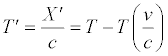The second term is a movement term, which can also be expressed by X(13a)

This - together with (13) - is the Voigt transformation, the predecessor of the Lorentz transformation .

How would a transformation look like if we assume, that both frames move in direction x towards or away from each other - similar to the Doppler Effect? We are searching for a common factor for both transformations that allows the simultaneous opposite movements of A and B in direction x.

X'=ɣ X (1-β) (13b)

X'=ɣ X (1+β) (14b)

We multiply and get

XX'=ɣ2 XX' (1-β2) (15)

And

ɣ=1/(16)

Here the ɣ-factor is also a geometric mean of the velocity parts ± v/c of A and B. In particular, it is reasonable for the case when the absolute velocities of A and B are not known.

From (13b) follows the Lorentz transformation

X'= ɣ(X-vT) (17)

And with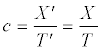T'= X'/c= ɣ [T-X (v/c2)]

The invariance of the space-time interval

Is the interpretation of the LT via the geometric mean actually justified? We regard the simplified equations of a spherical wave in the reference systems F and F' for a boost in direction x which follows from Einstein’s second postulate of relativity .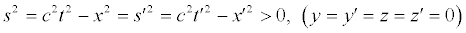(18)

It is now apparent that the geometric means s and s' are built by the sides of the rectangles of the space-time intervals a and b | a' and b' and an object that moves with a speed < c in space-time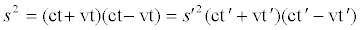(19)

a b a' b'

Shaping a rectangle with sides a and b into a square by retaining the same size of area, a side of this square is the geometric mean of sides a and b.

In this case one side of the square c2t2 resp. c2t'2 is linearly elongated to side a resp. to a' and the other side is linearly shortened to b resp. to b' but such that the areas s2 and s'2 remain the same. For that purpose one side of the square s2 must be multiplied by a stretching factor k and the other side by a compression factor 1/k: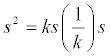(20)

Therefore,

a = ct + vt = ks (21)

b= ct –vt=(1/k)s⇒k/s=1/(ct-vt) (22)

As the invariance of areas s2 and s'2 is the basic condition we can also shrink side a and extend side b is the basic condition for the LT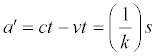(23)

b′ = ct + vt = ks (24)

As a b=a' b' and therefore a/a'=b'/b=k2 we get (besides the trivial result v=0)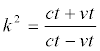(25)

Again, this is the formula of the Relativistic Doppler effect.

Geometrically the same areas s2 and s'2 are illustrated by the following graphic:

v=0 ks=ct + vt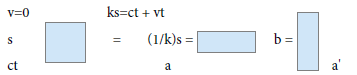Reference system F | F ' moves away from F | two possible interpretations |

The straightened up rectangle a'b' allows two interpretations

1) F moves away from F' with velocity –v or

2) F' approaches to F with velocity –v

Again, the common factor k is the formula of the Relativistic

Doppler effect but in this case a geometric mean due to the invariance of s2=s'2 . The second interpretation is invalid because F' cannot move back and forth at the same time. The first interpretation supports the results of the other two derivations of the LT: The frames A=F and B=F' must move in opposite directions simultaneously.

The above three derivations of the LT  were carried out by different methods. But the outcome is the same in each case and allows the following interpretation:

The Lorentz transformation is – like the Doppler effect - based on a frame outside of reference frames A and B, in which light propagates isotropically. The Lorentz-transformed physical values are geometric means  as a result of the simultaneous movement of frames A and B in opposite directions.

The advantage of this interpretation is that we can now understand the STR intuitively, but the consequences of this interpretation are significant.

Consequences

a) The geometric mean only makes sense if frames A and B can move against each other. This is not the case for example with experiments at CERN or with the gedankenexperiment in textbooks explaining the STR, where an observer on an embankment observes a train. It is also invalid to apply the LT to two fixed points on a rotating system, since they cannot move together.

b) The mean value is an estimate with an error that grows with the relative velocity v between A and B. However, at particle accelerators this velocity is very high. Therefore, the application of the LT cannot yield a reliable result.

c) The LT cannot be applied to distance X between frames A and B, as is done in various textbooks to explain the slower decay of muons at high velocities when they descend from a height of 10 km to Earth's surface. The invalidity of this approach is demonstrated in the following derivation of the length contraction of X:

B moves away from A (Galilean transformation):

X'=X (1-β) (13b)

B approaches A

X'=X (1+β) (13c)

=>X'2=X2(1-β2)

X'=(1/ɣ) X (14c)

This is the formula of the length contraction of X=c T where the distance x=vT between A and B is included. This is the result of the illogical assumption that B moves to A and moves away from A at the same time.

d) The application of the LT to sound should demonstrate the known relativistic effects near the speed of sound as well, but this is not the case.

e) The application of the LT to the Maxwell-equations results in the statement that light is transmitted isotropically in all reference frames:

c2t2 – r2=c2t'2 – r'2 =0 (18a)

The above considerations show that this is an incorrect mathematical construct based on a geometric mean. An isotropic light transmission is possible only if the light will be carried along with the light source. But this contradicts the second STR postulate which is experimentally verified. How could photons 'know' that their reference system was declared by a scientist as being at rest or as moving?

f) The Doppler Effect is based on the limited speed of sound or light. The observed changes of frequency are not real in the observed frame. In the Relativistic Doppler effect, the factor ɣ describes the situation that only the relative velocity v between A and B is known. In this case the relativistic change of frequency f is not real either in the observed system. As t=Nf, the time dilation is also not real. As the STR is based on the Relativistic Doppler effect k (Bondi) all relativistic effects are not real in the observed frame F'. The experimental findings however show that the mass increase and the time dilation (e.g. decay of muon) are real. Therefore, these findings must have another reason and cannot be caused by the LT.

#### Two Symmetry-Experiments

It has now become possible to test the above concept regarding the ɣ correction with the geometric mean.

1. Does an observed clock run faster?

If a car with clock A moves to a fixed clock B, what time difference would an observer in A measure in B? According to the rules of LT the observer in A can regard his frame at rest and B as a moving frame. The Hafele-Keating experiments  however show a slower running clock in its own system. In this case, A would measure a faster running clock in B, which is inconsistent with the STR. This experiment can now be demonstrated with new optical clocks.

2. Does the light clock really work?

Because of isotropic light emission, a laser pulse (in north-south direction) should hit a detector positioned exactly opposite in its own reference frame A [9-11]. Is this valid? Regarding isotropy as the result of a geometric mean real physics should show that the laser pulse would affect behind the detector with respect to the movement direction of A. This experiment can now be demonstrated with a squeezed laser. Such fundamental experiments are necessary to verify or disprove the LT as basis for the STR .

#### Conclusion

The STR experiments produce results that are in accordance with theory. This is akin to the Epicycle theory of the middle Ages, which accurately predicted the orbits of the planets. This was also a coordinate transformation without physical mechanisms. The STR shows that the classical physical formulas (containing space-, time-coordinates) must be multiplied by ɣ, but as discussed above this cannot be accomplished with the LT. Therefore, scientist should be open-minded and consider an unknown interaction between matter and an unknown medium. This should also solve the problem of the STR being incompatible with Quantum theory. Space-time modeling is useful for the time being but only a vague description of relativistic phenomena. However, lack of physical substantiation leads to a dead end in the long run. There must be a different explanation for gravity. Another option is to continue in position: Nobody can understand the STR/GTR and all seem to be happy.

#### References

Select your language of interest to view the total content in your interested language

### Article Usage

• Total views: 9494
• [From(publication date):
March-2016 - Feb 23, 2020]
• Breakdown by view type
• HTML page views : 9202Can't read the image? click here to refresh